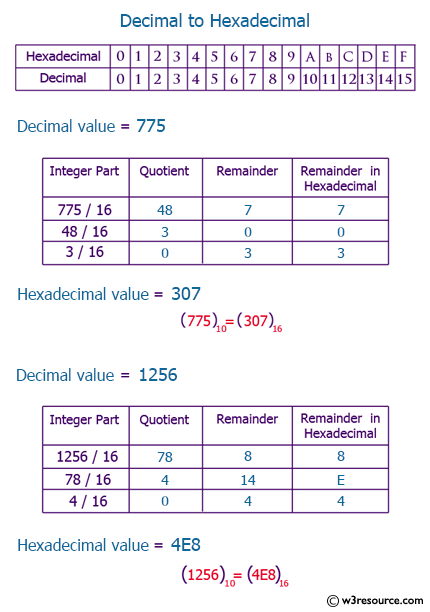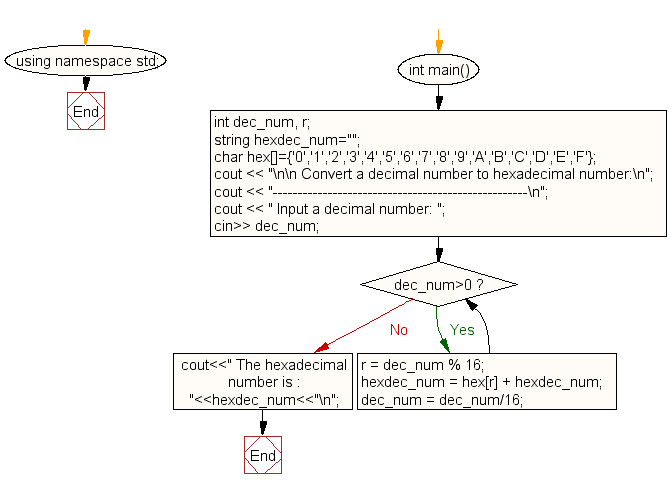﻿ C++ Exercises: Convert a decimal number to hexadecimal number - w3resource

# C++ Exercises: Convert a decimal number to hexadecimal number

## C++ For Loop: Exercise-71 with Solution

Write a program in C++ to convert a decimal number to a hexadecimal number.

Pictorial Presentation:Sample Solution:-

C++ Code :

``````#include <iostream>
#include <math.h>
using namespace std;

int main()
{
int dec_num, r;
string hexdec_num="";
char hex[]={'0','1','2','3','4','5','6','7','8','9','A','B','C','D','E','F'};
cout << "\n\n Convert a decimal number to hexadecimal number:\n";
cout << "---------------------------------------------------\n";
cout << " Input a decimal number: ";
cin>> dec_num;

while(dec_num>0)
{
r = dec_num % 16;
hexdec_num = hex[r] + hexdec_num;
dec_num = dec_num/16;
}
cout<<" The hexadecimal number is : "<<hexdec_num<<"\n";
}
``````

Sample Output:

``` Convert a decimal number to hexadecimal number:
---------------------------------------------------
Input a decimal number: 43
The hexadecimal number is : 2B
```

Flowchart:C++ Code Editor: